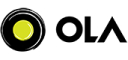New update is available. Click here to update.

# LRU Cache

Medium0/800 upvotes+2 more

## Problem Statement

##### Design a data structure that satisfies the constraints of a Least Recently Used (LRU).
``````1. Get(int num): If the key exists, it will return the value of the key stored. Else, return -1.
2. Put(int key, int value): If the key already exists, update the value of the key. Else add the key-value pair to the cache. If the number of keys is more than the capacity for this operation, delete the least recently key used.
``````
##### Example:
``````For the following input:

4 2
2 1 4
1 1
1 4

We will initialize an empty LRU cache for the first operation with a maximum capacity of 2.
For the first operation, we need to add a key-value pair (1,4) to the cache.
For the second operation, we need to return the value stored for key 1, i.e., 4
For the third operation, we need to return -1, as we don't have any key 4 in the cache.

So, the final output will be:
4  -1
``````
Detailed explanation ( Input/output format, Notes, Images )
##### Constraints -
``````1 ≤ T ≤ 10
1 ≤ N ≤ 10^5
ΣN ≤ 2*10^5
1 ≤ X ≤ (10^9)
1 ≤ Y ≤ (10^9)
1 ≤ A ≤ (10^9)
1 ≤ B ≤ (10^9)

Time Limit: 1 sec
``````
##### Sample Input 1 :
``````2
3 2
2 1 4
1 1
1 4
4 1
2 1 4
2 2 6
1 1
1 2
``````

##### Sample Output 1 :

``````4 -1
-1 6
``````
##### Explanation Of Sample Input 1 :
``````For the first case:

For the following input:

3 2
2 1 4
1 1
1 4

We will initialize an empty LRU cache for the first operation with a maximum capacity of 2.
For the first operation, we need to add a key-value pair (1,4) to the cache.
For the second operation, we need to return the value stored for key 1, i.e., 4
For the third operation, we need to return -1, as we don't have any key 4 in the cache.

So, the final output will be:
4  -1

For the second case:

For the following input:

5 1
2 1 4
2 2 6
1 1
1 2

We will initialize an empty LRU cache for the first operation with a maximum capacity of 1.
For the first operation, we need to add a key-value pair (1,4) to the cache.
For the second operation, we need to add a key-value pair (2,6) to the cache and remove (1,4) from the cache.
For the third operation, we need to return -1, as we don't have any key 1 in the cache.
For the fourth operation, we need to return the value stored for key 2, i.e., 6

So, the final output will be:
-1 6
``````
##### Sample Input 2 :
``````2
3 3
2 1 4
2 2 5
1 2
5 5
2 1 4
2 2 6
1 1
1 2
1 3
``````
##### Sample Output 2 :
``````5
4 6 -1
``````AutocompleteConsole